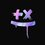# Unsolved Questions - 2

The repetition.
I think that you might have heard of this problem earlier, it's an equation which you are supposed to do repeatedly. All you have to do is this;
- Take any even number, if it's even divide it by 2 - But if it's odd, multiply it by 3 and add 1
For those who doesn't know about this, try it yourselves! Keep repeating this process and see the final result (there is no "final" result actually). I will give you a few examples;
#15
15 -> 46 -> 23 -> 70 -> 35 -> 106 -> 53 -> 160 -> 80 -> 40 -> 20 -> 10 -> 5 -> 16 -> 8 -> 4 -> 2 -> 1 -> 4 -> 2 -> 1... And so on
Let's see the final stop before the repitition begins with another example.
#23
23 -> 70 -> 35 -> 106 -> 53 -> 160 -> 80 -> 40 -> 20 -> 10 -> 5 -> 16 -> 8 -> 4 -> 2 -> 1 -> 4 -> 2 -> 1 -> 4...
Then again it was one wasn't it?
Mathematicians haven't found a particular equation for this as yet, but can you figure out one? I'm trying to do so, but I'm not a mathematician myself to find one! If you can come up with one, drop a text below! ;)Note by Hasna Hassen
3 months, 3 weeks ago

This discussion board is a place to discuss our Daily Challenges and the math and science related to those challenges. Explanations are more than just a solution — they should explain the steps and thinking strategies that you used to obtain the solution. Comments should further the discussion of math and science.

When posting on Brilliant:

• Use the emojis to react to an explanation, whether you're congratulating a job well done , or just really confused .
• Ask specific questions about the challenge or the steps in somebody's explanation. Well-posed questions can add a lot to the discussion, but posting "I don't understand!" doesn't help anyone.
• Try to contribute something new to the discussion, whether it is an extension, generalization or other idea related to the challenge.

MarkdownAppears as
*italics* or _italics_ italics
**bold** or __bold__ bold
- bulleted- list
• bulleted
• list
1. numbered2. list
1. numbered
2. list
Note: you must add a full line of space before and after lists for them to show up correctly
paragraph 1paragraph 2

paragraph 1

paragraph 2

[example link](https://brilliant.org)example link
> This is a quote
This is a quote
    # I indented these lines
# 4 spaces, and now they show
# up as a code block.

print "hello world"
# I indented these lines
# 4 spaces, and now they show
# up as a code block.

print "hello world"
MathAppears as
Remember to wrap math in $$ ... $$ or $ ... $ to ensure proper formatting.
2 \times 3 $2 \times 3$
2^{34} $2^{34}$
a_{i-1} $a_{i-1}$
\frac{2}{3} $\frac{2}{3}$
\sqrt{2} $\sqrt{2}$
\sum_{i=1}^3 $\sum_{i=1}^3$
\sin \theta $\sin \theta$
\boxed{123} $\boxed{123}$

Sort by:

Very intriguing! You've probably already seen this, but for anyone who hasn't, here is a summary of what is currently known about this process.

- 3 months, 3 weeks ago

Hmmm... The theory is alot much complicated than I thought it would be!

- 3 months, 2 weeks ago